### Home > CALC > Chapter 6 > Lesson 6.1.1 > Problem6-10

6-10.
1. Rewrite the following as powers of the specified base. An example is shown below. Homework Help ✎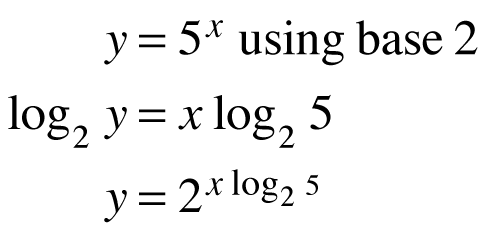1. y = 3x using base 5

2. y = 7x using base 10

3. y = 2x using base e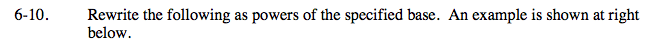Use the example to the right to help you with these problems.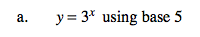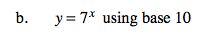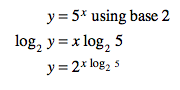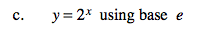ln(y) = x ln(2)
y = ex ln(2)